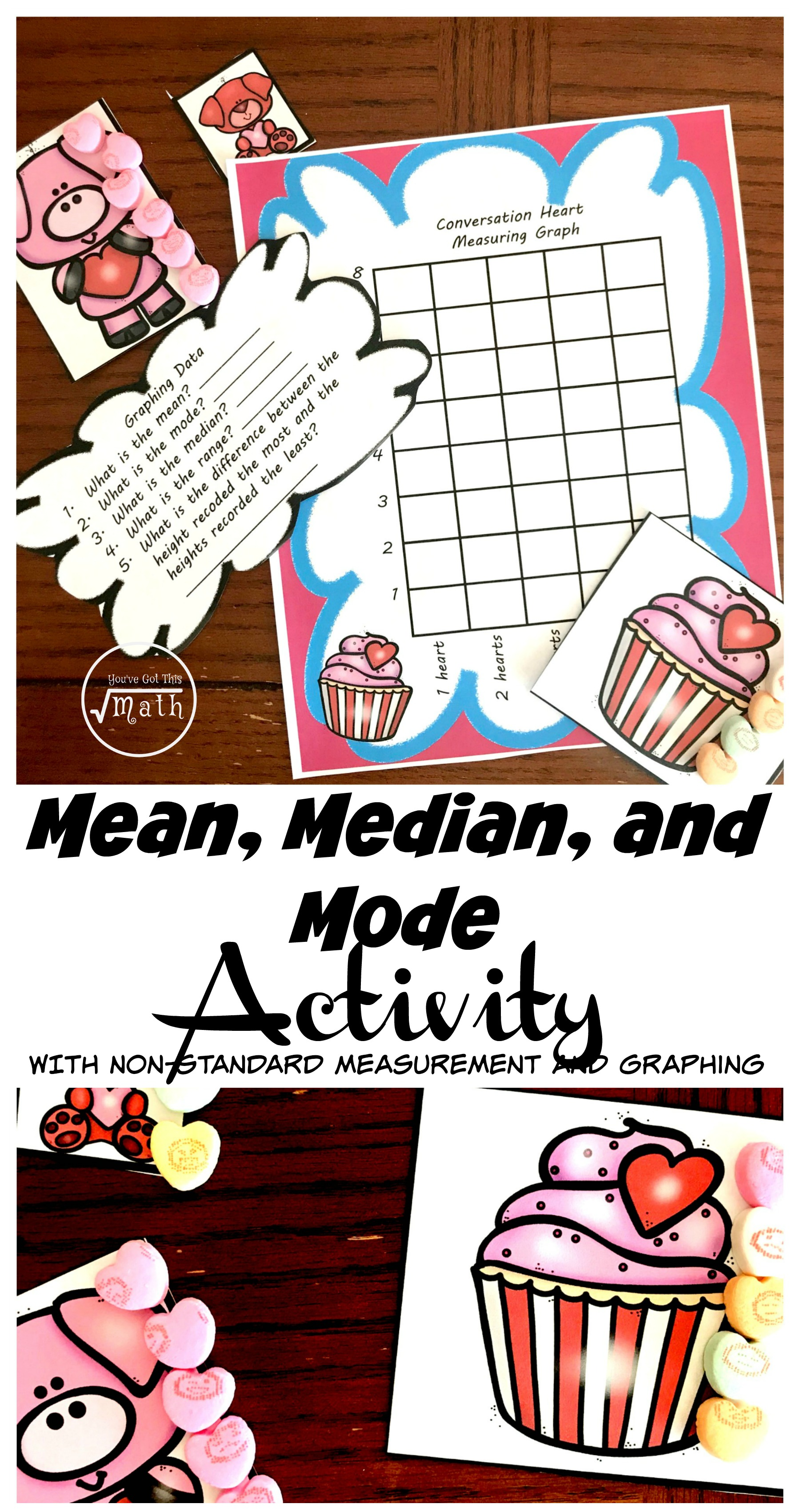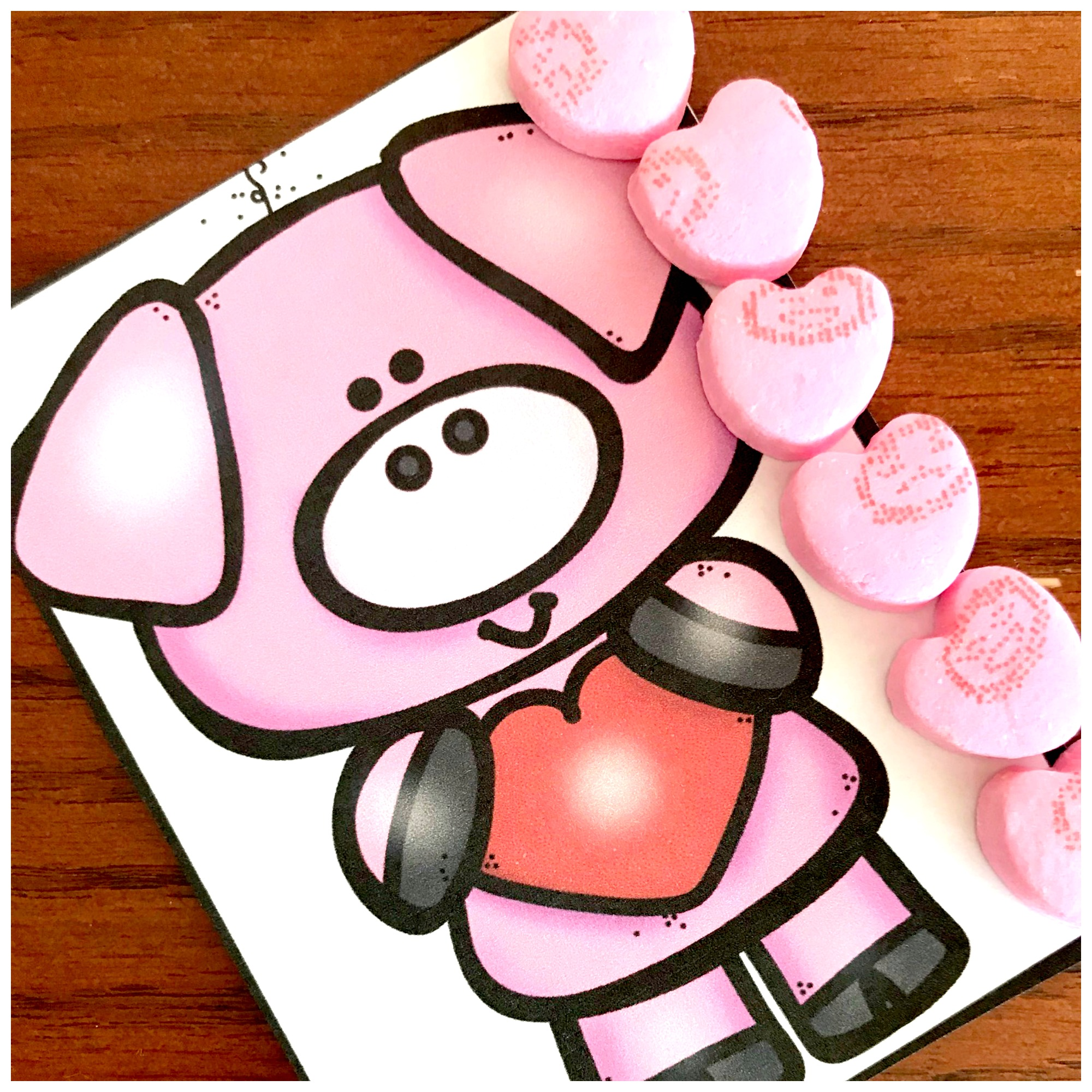Home » Math » Find the Mean, Median, and Mode with this Fun, Hands-on Activity

# Find the Mean, Median, and Mode with this Fun, Hands-on Activity

Practice non-standard measurement and graphing with this free Valentine’s Day Printable. Then find the mean, median, and mode based on the measurement data.

You can get this free printable by clicking on the blue button at the bottom of the post.This post contains affiliate links.

THere are so many layers to teaching measurement. There is non-standard measurement, measuring with rulers, conversions, graphing, and finding the mean, median, and mode.

With this activity, you get to practice non-standard measurement, graphing, and finding the mean, median, and mode.

Get 30 of My Favorite Measurement Activities Here## Prep -Work For Find the Mean, Median, and Mode Activity

• First, print off pictures on card stock paper.
• Next, cut out and laminate.
• Print off the graph and questions on regular copy paper.
• Finally, provide conversation hearts and pencils.

## Directions –

There are a few different levels of this activity, which makes it suitable for various age groups. Simply choose what steps your kiddos do depending on their math levels or age.

#### Non-Standard Measurement

This step is obviously the easiest step and one even some preschoolers can conqueror. All our kiddos have to do is measure each Valentine picture with conversation hearts.#### Graphing

As we move to the next step, the level of difficulty increases. Now the children need to graph how many hearts were used to measure each picture.

In this example, six hearts were used. Therefore, the students would color in a space above six hearts.

#### Finding the Mean, Median, and ModeNow we jump up one more level of difficulty.

After the graph is completed, it is time to work on finding the mean, median, and mode. So how do we do this?

First children must understand the graph. They must remember that each time they colored in a box that they are saying they have one picture that equals that amount.

Looking at this graph, the numbers we would use to find the mean, median, and mode are as follows.

3, 3, 3, 4, 5, 5, 5, 6, 8, 9So how do we find all those M’s for these numbers?

Here we go….

#### Mean

Mean is another word for average, and this one requires the most work. The first step is to add up all the numbers.

In our example, our sum is 51.

The next step is to divide by the numbers you added up….which is 10. So 51 ÷ 10 = 5 with a remainder of 1. We will just say that the mean is 5.

#### Median

So our next word is median. This is a pretty easy one. Which number is directly in the middle. One way our kiddos can figure this out is to cross off numbers on each side until is there is one left in the middle.

In our example, we end up with two fives. SO we have to find the average or mean fo those two numbers….5 + 5 = 10 and 10 divided by 2 is 5. So the median is 5.

#### Mode:

Easiest one!! Just like Mode starts with “mo” so does Most. All your kiddos have to do is find the number that is used the most. In our case, we have two modes…3 and 5.

Enjoying having some Valentine fun while finding the mean, median, and mode.

You’ve Got This

Rachel

Get my other conversation heart activities here!